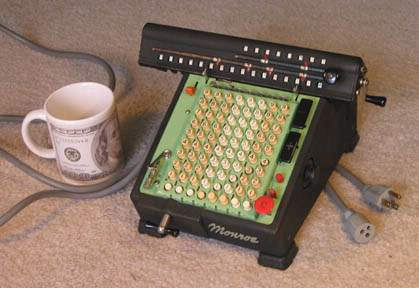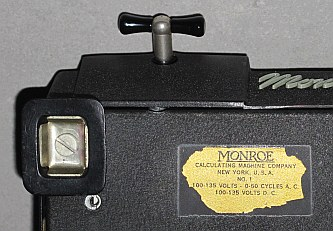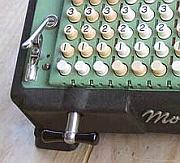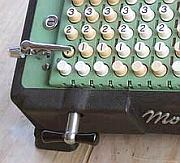previous <<==>> next

 MONROE   Model-1 Have a look at an older model and a newer version``` TECHNICAL DATA of the Electro-Mechanical Calculator MONROE »Model-1« *************************************************************************** Model: LA5-160 Serial Number: 416982 ( green/black ) Dimensions: (ca.) Width = 11_1/2" / 29 cm Depth = 11 " / 28 cm Height = 6_1/2" / 17 cm Weight: (ca.) 14 lbs / 6.5 kg Power Supply: 110 - 135 V AC / DC Mechanics: 8*10 Full-Keyboard Split-Stepped Drum / Geteilte Staffelwalze  10s Carry Mechanism Counter Stepping Mechanism + Animation Functions: Add, Subtract, Multiply, Divide Division-Automatic! Registers: Input = 8 Decimals Counter = 8 Decimals (NO Carry; Neg.Figures RED) Arithmetic = 16 Decimals Manufacturer: MONROE Calculating Machine Co. New York / USA 1950 H O W T O U S E the MONROE »Model-1« ******************************************** BEFORE STARTING A TASK: ----------------------- RELEASE the red REPEAT-Button (R) by pressing the red button () above. CLEAR the KEYBOARD by pressing the lower right red Button (0). CLEAR the COUNTER unit by turning the carriage crank clockwise. CLEAR the ARITHMETIC unit by turning the same crank counter-clockwise. Lift and shift the CARRIAGE to the far left ( = POSITION 1). ADDITION & SUBTRACTION: ----------------------- Example: 123 + 45 - 6 = 162 ADD: Enter the first number (123) in the far right columns. Press the PLUS-key (+) to transfer the number into arithmetic unit. The counting unit displays the figure 1. Enter the second number (45). Press the PLUS- key (+) to add the number. The arithmetic unit displays the intermediate sum (168) and the counting unit displays the figure 2. SUBTRACT: Enter the third number (6). Press the MINUS-key (-). The arithmetic unit displays the result (162) and the counting unit is decreased by 1. REMARK: NEGATIVE RESULTS are displayed in the arithmetic unit as the COMPLEMENT of the next higher 10, 100, 1000, ... Example: -12 = 99...9988 MULTIPLICATION: --------------- Example: 123 x 45 = 5535 Clear counter and arithmetic units. Press the right red REPEAT-Button (R). Enter the multiplicand (123) in the far right columns. The multiplicator (45) has two digits, so the arithmetic unit is moved to position 2 with a clockwise turn of the T-Handle in front. Repeat pressing the PLUS-key (+), until the first figure of the multiplicator (4) will appear in the 2nd position of the counter unit. Move the arithmetic unit with the T-Handle to position 1. Repeat pressing the PLUS-key (+), until the second figure of the multiplicator (5) appears in the 1st position of the counter unit. The multiplication is done: The multiplicand (123) stays in keyboard, the multiplicator (45) is in the counter unit and the result (5535) is in the arithmetic unit. DIVISION: --------- Example: 22 : 7 = 3.1428571 Remainder 3 Division requires 3 steps: (A) To Set the Dividend into Arithmetic Unit: ----------------------------------------- For the maximum number of decimals, pull out the arithmetic unit to the far right position. Enter the dividend (22) in the far right columns. Press the PLUS-key (+) to transfer into arithmetic unit. (B) To Set the Divisor in the Keyboard: ----------------------------------- Clear the counter unit by turning the carriage crank clockwise. Enter the divisor (7) below the dividend (22). Press the right red REPEAT-Button (R). (C) To Divide: ---------- Set the upper left red toggle lever to divide (:) and the machine will calculate all decimals (and moves the carriage) automatically. The result (3.1428571) is in the counter unit ( in RED ), and the remainder (3) is in the arithmetic unit. The divisor (7) stays in the input unit, therefore an additional decimal can be estimated ... ( 4, cause 4 x 7 = 28). Have a look at "Calculating Trickies" ... R E M A R K T O » M O D E L - 1 « vs. » Model LA5-160 « *************************************************************************** The LABEL on the back side is naming this calculator " NO. 1 " ...It is the same label as the one under the OLD » MODEL-1 « - MY GUESS: Because of the commercial success of the OLD MODEL-1, the MONROE Company continued to use this "BRAND-MARK" also for their newer versions ... R E M A R K S to the missing / restored "ITEM-COUNTER-CLIP": ===========================================================================Because the counter has no 10s-carry, there is no "build-in" counting of the items to add. Here the "Item-Counter-Clip" is useful: Home-made out of an "Aligator Clip" - When this clip is switched to the right the (1) key is pressed, the arithmetic unit will count the items in this column(s) ... In this case the "CLEAR KEYBOARD" Button will NOT clear this (1) key. Have a look at the HOME-MADE POWER PLUG for 220 VAC ... PS.: The Picture on the 100 US\$ Note is Benjamin Franklin impressum: ************************************************************************** © C.HAMANN http://public.BHT-Berlin.de/hamann 12/15/08 ```